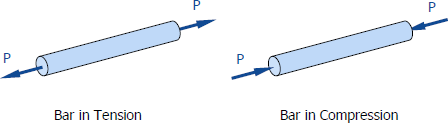# Normal Stresses

Normal stress will occur to members that are axially loaded. Axial force can be tension or compression and applied at the centroid of cross-section of the member.

$\sigma = \dfrac{P}{A}$

where P is the applied normal load in Newton and A is the area in mm2. The maximum stress in tension or compression occurs over a section normal to the load.

Normal stress is either tensile stress or compressive stress. Members subject to pure tension (or tensile force) is under tensile stress, while compression members (members subject to compressive force) are under compressive stress.Compressive force will tend to shorten the member. Tension force on the other hand will tend to lengthen the member.